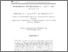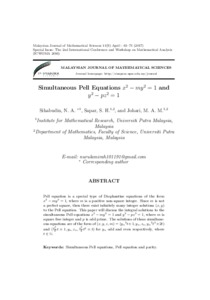# Simultaneous pell equations x²-my² = 1 and y²-pz² = 1

## Citation

Sihabudin, Nurul Amirah and Safar, Siti Hasana and Johari, Mohamat Aidil (2017) Simultaneous pell equations x²-my² = 1 and y²-pz² = 1. Malaysian Journal of Mathematical Sciences, 11. 61 - 71. ISSN 1823-8343; ESSN: 2289-750X

## Abstract

Pell equation is a special type of Diophantine equations of the form x²−my²= 1, where m is a positive non-square integer. Since m is not a perfect square, then there exist infinitely many integer solutions(x, y)to the Pell equation. This paper will discuss the integral solutions to the simultaneous Pell equationsx²−my²= 1 and y²−pz²= 1, where m is square free integer and p is odd prime. The solutions of these simultaneous equations are of the form of(x, y, z, m) = (yn²t±1, yn, zn, yn²t²±2t)and(y²n/²t±1, yn, zn, y²n/4t²±t) for yn odd and even respectively, where t ∈ N.Preview
Text
Simultaneous pell equations x²-my² = 1 and y²-pz² = 1.pdfView Item